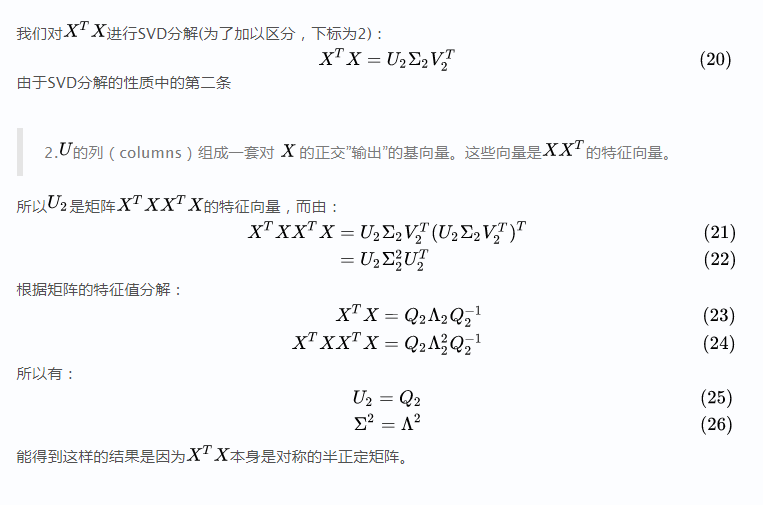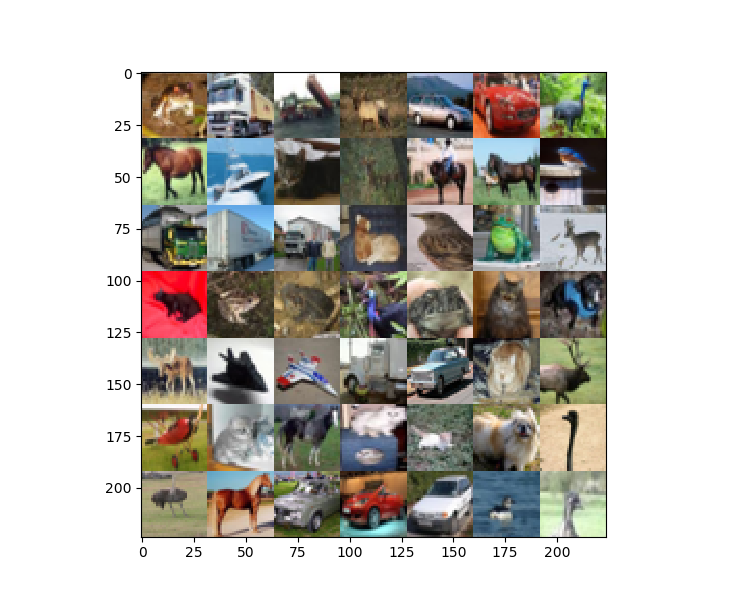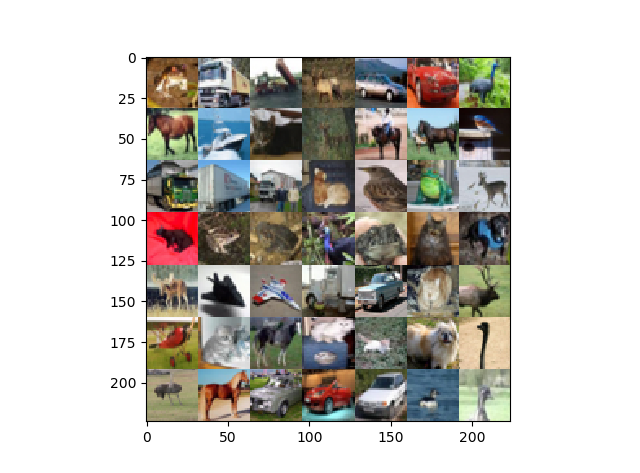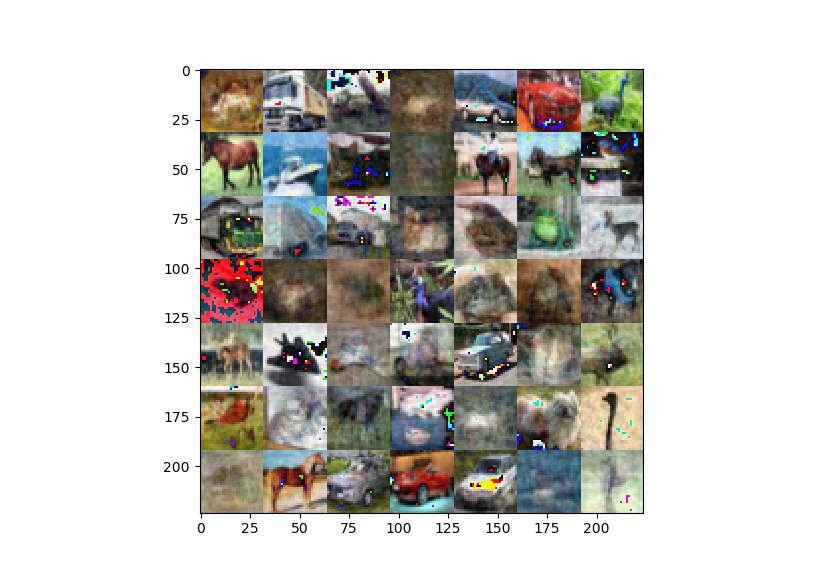## 降维

pca的实现思路也有两种解释：
1、第一种是最大可分性：使得投影点在超平面上的投影尽可能分开
2、第二种是最近重构性：使得样本点到超平面的距离都足够近

1、对数据标准归一化（去均值，各维度统一方差，将各维度在统一尺度上计算）
2、计算协方差矩阵 C=1/(m1)XTX $C=1/(m-1)X^{T}X$
3、计算C的特征向量矩阵和特征值，将特征值对应的特征向量按照特征值由大到小排列得到特征向量矩阵，取前k列特征向量组成投影矩阵 Ureduce $U_{reduce}$
4、计算降维后的数据 Xnew=XUreduce $X_new=X*U_{reduce}$
5、这样就将n维的矩阵降到k维

SVD（奇异值分解）是对矩阵分解的一种方法， X=USVT $X=USV^{T}$

1、对X进行svd，得到的V（ XTX $X^{T}X$的特征向量矩阵）就是C对应的特征向量矩阵，由于svd得到的奇异值正好是从大到小进行排列，那么这儿得到的U正好是与特征值大小顺序对应构成的特征向量。为什么V是C的Q（特征向量矩阵）呢？

X=USVT $X=USV^{T}$

C=XTX=USVTTUSVT=VS2VT=VS2V1 $C=X^{T}X=（USV^{T}）^{T}USV^{T}=VS^{2}V^{T}=VS^{2}V^{-1}$

V=Q,=S2 $V=Q,\bigwedge=S^{2}$

2、对 XTX $X^{T}X$进行svd，得到的U就是X的特征向量矩阵。2017.11.24更新———————————————–

import matplotlib.pyplot as plt
import numpy as np

def unpickle(file):
import pickle
with open(file, 'rb') as fo:
dict = pickle.load(fo, encoding='bytes')
return dict

def show(imgs):
matrix=np.zeros(shape=(7*32,7*32,3),dtype=np.uint8)
print(matrix.shape)
print(imgs.shape)
index=-1
for i in range(7):
for j in range(7):
index+=1
matrix[i*32:(i+1)*32,j*32:(j+1)*32,:]=imgs[index]
plt.imshow(matrix)
plt.show()

def pca(imgs_raw):
'''
:param imgs: 49*3072
:return:
'''
imgs_raw=imgs_raw.astype(np.float32)
imgs=imgs_raw-np.mean(imgs_raw,axis=0)
cov=np.dot(imgs.T,imgs)/(imgs.shape*imgs.shape)

U,S,V=np.linalg.svd(cov)

# 去相关
#     imgs=np.dot(imgs,U)

# 降维
imgs_pca=np.dot(imgs,U[:,:30])

imgs_pca_r=np.dot(imgs_pca,U[:,:30].T)
imgs_pca_r+=np.mean(imgs_raw,0)
imgs_pca_r = imgs_pca_r.reshape((len(imgs), 3, 32, 32)).transpose(0, 2, 3, 1)
show(imgs_pca_r)
# imgs = imgs.reshape((len(imgs), 3, 32, 32)).transpose(0, 2, 3, 1)

if __name__ == '__main__':
data_labels=unpickle('H:\\Machine Learning\\'
'deep-learning-master\\deep-learning-master\\image-classification\\cifar-10-batches-py\\data_batch_1')
imgs=data_labels[b'data'][:49]
pca(imgs)
# show(imgs)